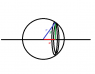# Hollow Sphere Moment of Inertia

GeneralOJB
I need to find the moment of inertia of a sphere of radius ##r## and mass ##m## about an axis through it's centre. I've already done it and got the correct answer of ##\frac{2}{3}mr^2## however I have tried doing it using a different method to see if I get the same answer, but I don't, and I don't know why.

See the image attached.

The moment of inertia of one of the hoops is ##\displaystyle\frac{m}{4\pi r^2}\times 2\pi y \delta x\times y^2##.

##x^2+y^2=r^2## so I just need to evaluate ##\displaystyle\int_{-r}^r \frac{m}{4\pi r^2}\times 2\pi (r^2-x^2)^{3/2}\,\mathrm{d}x##

But I don't get the right answer.

#### Attachments

•Untitled.png
1.8 KB · Views: 1,171
Last edited:

dauto
There is no image attached

dauto
Seems like your expression for the hoop moment of inertia is wrong. It should be $$\frac{m}{4\pi r^2}\times 2\pi y \sqrt{(\delta x)^2+(\delta y)^2} \times y^2$$

GeneralOJB
Sorry, I forgot to add it. It's just showing what my x,y, and r mean.

Wouldn't the width of the hoop just be ##\delta x## ?

dauto
Yes, but the mass of the hoop is proportional to its surface area which depends on both δx and δy.

dauto
just imagine for a second a hoop where δx is zero, but δy isn't. You get that when calculating the Moment of inertia of a disc. The expression you had is good for hoops taken from a cylinder, but not for hoops taken from a sphere

GeneralOJB
Ah, I understand. This method won't work then, the previous one involved ##r\delta\theta##.

For the derivation of the moment of inertia of a sphere that isn't hollow, the disc is assumed to have volume ##\pi y^2 \delta x##. Why is this ok?

dauto
Ah, I understand. This method won't work then, the previous one involved ##r\delta\theta##.

For the derivation of the moment of inertia of a sphere that isn't hollow, the disc is assumed to have volume ##\pi y^2 \delta x##. Why is this ok?

The method works, but you first must find an expression for δy by implicitly differentiating the expression for r2, and setting it to zero since r is constant. The other method you describe is using the volume of a disc, not its surface area.

GeneralOJB
I know, but I'm wondering why it's OK to approximate the width as ##\delta x## to find the volume, but not to find the surface area.

dauto
δx is the width of the slice, so that is the correct factor for the volume (not an approximation). The slice doesn't reach the surface of the sphere at a right angle, so the width of the ribbon taken by the intersection of the disc with the sphere's surface is different from the with of the slice itself.

GeneralOJB
How is it not an approximation? A slice of a sphere isn't cylindrical, it has some curvature.

dauto
How is it not an approximation? A slice of a sphere isn't cylindrical, it has some curvature.

It's an infinitesimal slice

GeneralOJB
It's an infinitesimal slice

In the case of the hollow sphere, the ribbon would also be an infinitesimal slice, so I don't really understand why ##\delta x## wouldn't work in that case, when the approximation(which it is, at least before the limit is taken) works in the case of the solid sphere. I'm not understanding the difference between the two cases.

dauto
The difference is that in one of them you're calculating the volume and the shape of the edge doesn't matter. In the other you are calculating the area of the edge and its shape is crucial.

smarty girl
Anyone can te me the final expression of moment of inertia of hollow sphere??

Gold Member
2022 Award
I don't know, whether this is a (simple) homework question, but here's how to calculate it. We introduce spherical coordinates, and we calculate the moment of inertia around the polar axis. Then you get
$$\Theta=\int_0^{\pi} \mathrm{d} \vartheta \int_0^{2 \pi} \mathrm{d} \varphi \frac{M}{4 \pi r^2} r^4 \sin^3 \vartheta=\frac{M r^2}{4 \pi} \int_0^{\pi} \mathrm{d} \vartheta \int_0^{2 \pi} \mathrm{d} \varphi \sin^3 \vartheta.$$
Now you should evaluate the integral yourself. Hint: substitute ##u=\cos \vartheta##, ##\mathrm{d} u = \mathrm{d} \vartheta \sin \vartheta##.

hackhard
The moment of inertia of one of the hoops is (m/4.pi.r^2)*(2.pi.y.dx)*(y^2)
no it is not because
surface area of hoop element= slant height * circumference
(not vertical height * circumference , as in your expression)
so moment of inertia of one of the hoops
=(m/4.pi.r^2)* ( dx . sec(t) . 2.pi.y) * (y^2) (where t is angle bet axis of hoop and line parallel to slant side surface of hoop)
tan(pi-t)= (-x) / ((r^2-x^2)^0.5)
so tan(t)= (x) / ((r^2-x^2)^0.5)
so sec (t) can be found
final exp for moment of inertia of one of the hoops = (m * (r^2 - x^2) * dx) / (2* r)
integrate from -r to r you get (2/3)m(r^2)
piece of cake